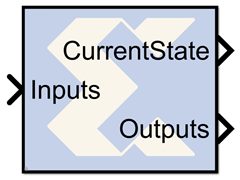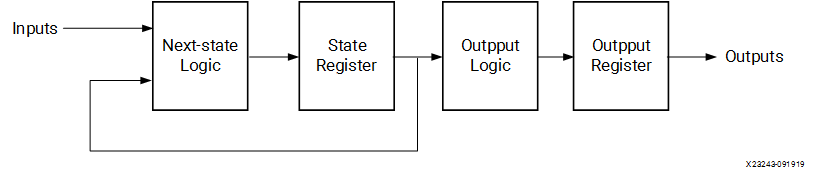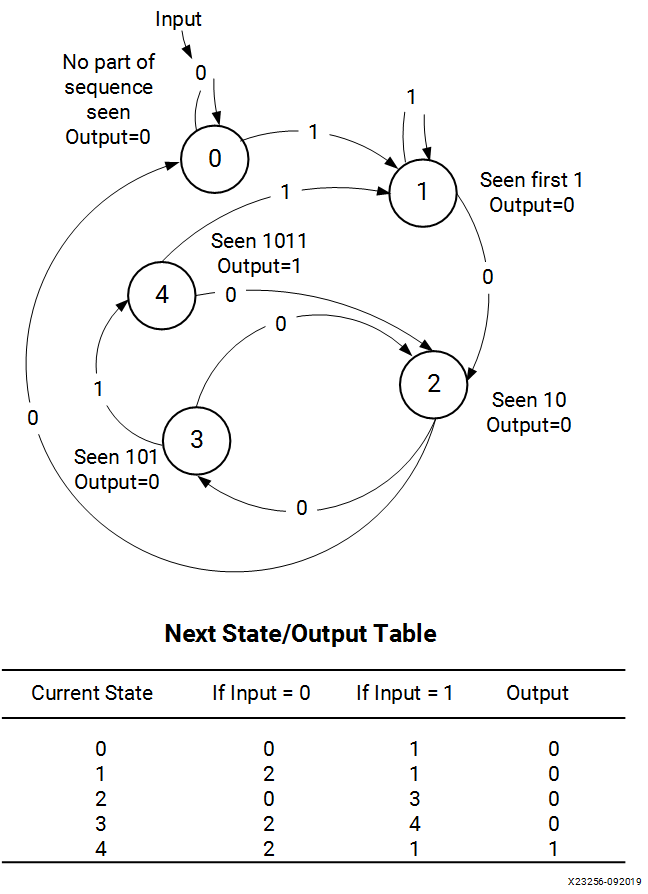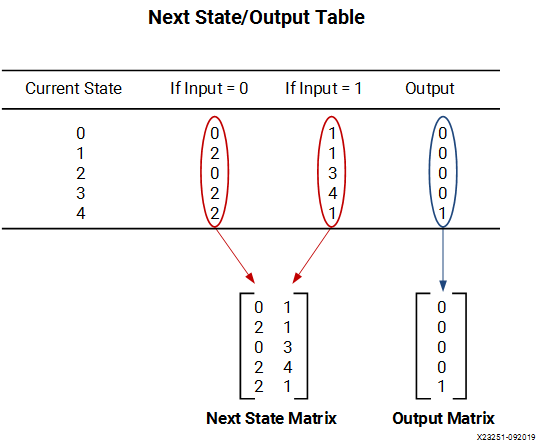# Registered Moore State Machine - 2020.2 English

## Vivado Design Suite Reference Guide: Model-Based DSP Design Using System Generator (UG958)

Document ID
UG958
Release Date
2020-11-18
Version
2020.2 English

A "Moore machine" is a finite state machine whose output is only a function of the machine's current state. A "registered Moore machine" is one having registered output, and can be described with the following block diagram:Figure 1. Moore State Machine Block DiagramThere are many ways to implement such state machines in System Generator, e.g., using the Mcode block. This reference block provides a method for implementing a Moore machine using block and distributed memory. The implementation is very fast and efficient. For example, a state machine with 8 states, 1 input, and 2 outputs that are registered can be realized with a single block RAM that runs at more than 150 MHz in a Xilinx Virtex device.

The transition function and output mapping are each represented as an N x M matrix, where N is the number of states, and M is the size of the input alphabet (e.g., M = 2 for a binary input). It is convenient to number rows and columns from 0 to N – 1 and 0 to M – 1 respectively. Each state is represented as an unsigned integer from 0 to N - 1, and each alphabet character is represented as an unsigned integer from 0 to M - 1. The row index of each matrix represents the current state, and the column index represents the input character.

For the purpose of discussion, let F be the N x M transition function matrix, and O be the N x M output function matrix. Then F(i,j) is the next state when the current state is i and the current input character is j, and O(i,j) is the corresponding output of the Mealy machine.

## Example

Consider the problem of designing a Moore machine to recognize the pattern '1011' in a serial stream of bits. The state transition diagram and equivalent transition table are shown below.

Figure 2. Transition TableThe table lists the next state and output that result from the current state and input. For example, if the current state is 4, the output is 1 indicating the detection of the desired sequence, and if the input is 1 the next state is state 1.

The Registered Moore State Machine block is configured with next state matrix and output array obtained from the next state/output table discussed above. They are constructed as shown below:

Figure 3. Next State Output TableThe rows of the matrices correspond to the current state. The next state matrix has one column for each input value. The output array has only one column since the input value does not affect the output of the state machine.

## Block Parameters

The block parameters dialog box can be invoked by double-clicking the icon in your Simulink model.

The next state logic and state register in this block are implemented with high speed dedicated block RAM.

The number of bits used to implement a Moore state machine is given by the equations:

ds = (2k)(2i) = 2k+i

ws = k

Ns = ds*ws = (k)(2k+i)

where

Ns = total number of next state logic block RAM bits

s = number of states

k = ceil(log2(s))

i = number of input bits

ds = depth of state logic block RAM

ws = width of state logic block RAM

The following table gives examples of block RAM sizes necessary for various state machines:

Table 1. State Machine block RAM Requirements
Number of States Number of Input Bits Block RAM Bits Needed
2 5 64
4 1 8
8 6 1536
16 5 2048
32 4 2560
52 1 768
100 4 14336

The block RAM width and depth limitations are described in the core datasheet for the Single Port Block Memory.